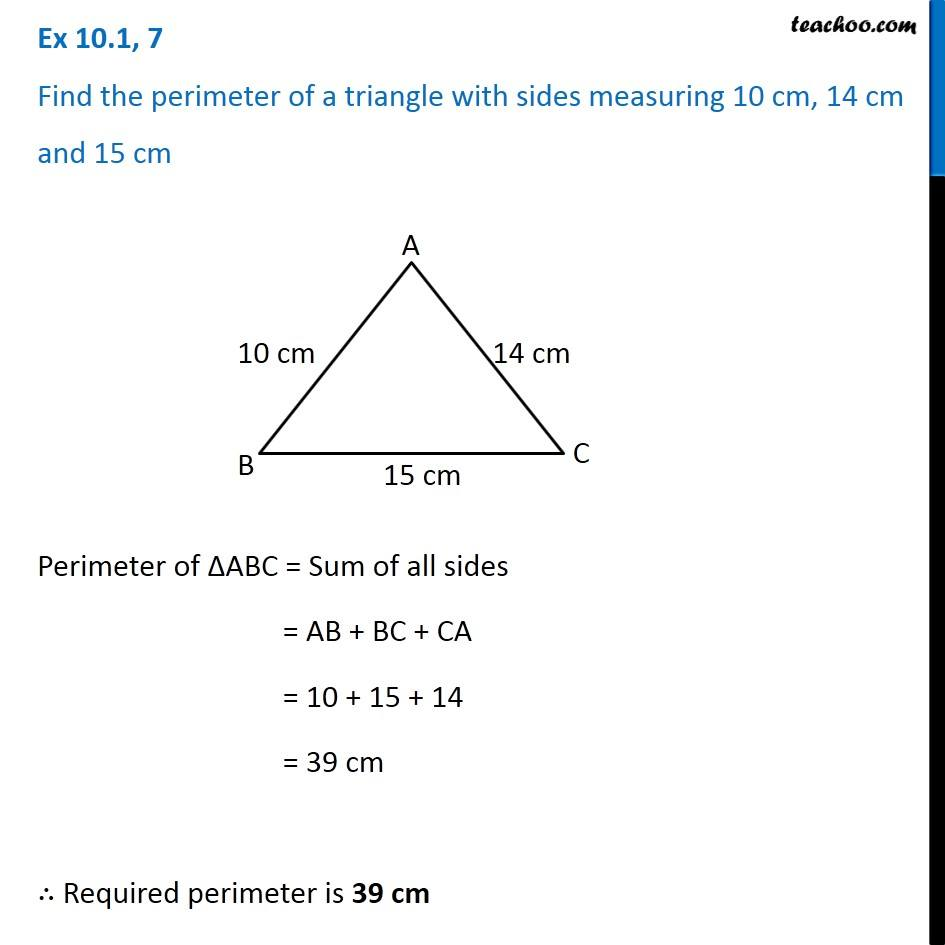Subscribe to our Youtube Channel - https://you.tube/teachoo

1. Chapter 10 Class 6 Mensuration
2. Concept wise
3. Perimeter of Triangle

Transcript

Ex 10.1, 7 Find the perimeter of a triangle with sides measuring 10 cm, 14 cm and 15 cm Perimeter of ∆ABC = Sum of all sides = AB + BC + CA = 10 + 15 + 14 = 39 cm ∴ Required perimeter is 39 cm

Perimeter of Triangle